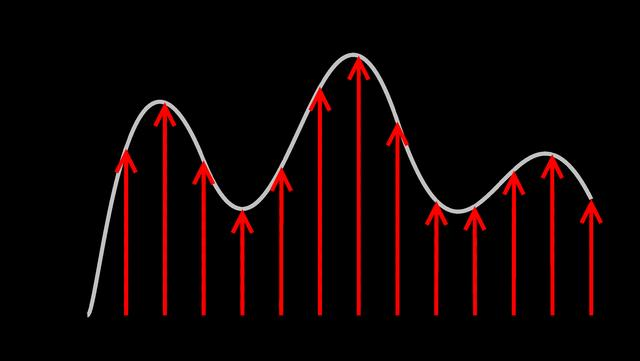Home Technology Sampled signal

# Sampled signal

## Spectrum continuation

Example 1

The Fourier transform of the ideal sampled signal can prove that the spectrum of the ideal sampled signal is the periodic extension of the spectrum of the continuous signal. The repetition period is Ws (sampling frequency), that is,

Example 2

where is the frequency spectrum of the ideal sampling signal, which is the Fourier transform of the continuous signal. Obviously, it is a continuous function of frequency Ω.

Digital corner frequency.

## Sampling theorem

If the signal xa(t) is a real-band limited signal, and the highest frequency spectrum does not exceed Ws/2, that is

the sampling theorem

Then in the ideal sampling spectrum, the baseband spectrum and the harmonic modulation spectrum of each order do not overlap each other. An ideal low-pass filter with a bandwidth of Ws/2 can filter out the harmonic modulation spectrum of each order. , To retain the undistorted baseband spectrum, so as to restore the original continuous signal without distortion.

hundieIf the highest frequency spectrum of the signal exceeds Ws/2, then in the ideal sampled spectrum, the modulation spectrums of each time will overlap each other, and there will be a phenomenon of “mixing” of the spectrum, such as Figure 1.4 shows the aliasing diagram of the spectrum. For the sake of brevity, the aliasing diagram of the spectrum in Figure 1.4 treats Xa(jW) as a scalar. Generally, Xa(jW) is a complex number, and the overlap is also the addition of complex numbers. When spectrum aliasing occurs, it is generally impossible to filter out the baseband spectrum without distortion, and the signal recovered by baseband filtering will be distorted.

Therefore, half of the sampling frequency, Ws/2, is called the folding frequency. It is like a mirror. When the signal spectrum exceeds it, it will be folded back, causing spectrum confusion.

Nyquist sampling theorem: In order to restore the real signal without distortion after sampling, the sampling frequency must be greater than twice the highest frequency of the signal, that is, Ωs≥2Ωmax.

In actual work, in order to avoid spectrum aliasing, the sampling frequency is always selected to be larger than twice the highest frequency Wmax of the signal, such as Ws>(3~5)Wmax. At the same time, in order to prevent the spurious spectrum higher than the folding frequency from entering the sampler and causing spectrum aliasing, a protective pre-low pass filter (anti-aliasing filter) is often added before the sampler to prevent frequency components higher than WS/2 from entering .

3) Laplace transform of the sampled signal

After ideal sampling, the Laplace transform of the signal is periodically extended along the imaginary axis on the S plane, that is, the imaginary The axis is a periodic function.

In the field of information processing, sampling signal means that the analog signal is first sampled by a sampler at a certain time interval to obtain a discrete signal in time.

These signals are also discretized numerically by the analog-to-digital converter (ADC), so as to obtain a digital signal that is both numerically and temporally discrete.

The whole process is called sampling. In many cases, "sampling" refers to the process of combining sampling and quantization.

Sampling, also called sampling, is the discretization of the signal in time, that is, the instantaneous value of the analog signal x(t) is taken point by point according to a certain time interval △t. It is realized by multiplying the sampling pulse and the analog signal.

Sampling interval selection and signal confusion: To sample an analog signal, first determine the sampling interval. How to choose △t reasonably involves many technical factors that need to be considered. Generally speaking, the higher the sampling frequency, the denser the number of sampling points, and the closer the resulting discrete signal is to the original signal. However, too

High sampling frequency is not advisable. For signals of fixed length (T), too much data volume (N=T/△t) is collected, which adds unnecessary calculation work to the computer If the amount of data (N) is limited, the sampling time is too short, which will cause some data information to be excluded. Sampling

The frequency is too low and the sampling point interval is too far, the discrete signal is not enough to reflect the original signal waveform characteristics, and the signal cannot be restored, causing signal confusion.

Intuitively speaking, signal aliasing is to mistake signals that should be high-frequency signals for low-frequency signals.